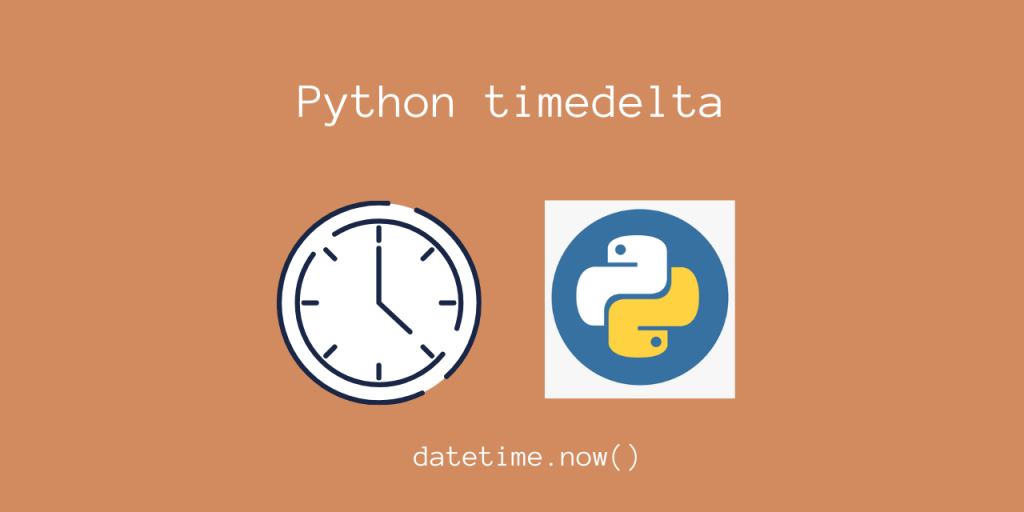# How to Work with Python TimeDelta?Hello everyone! In today’s article, we’ll be taking a look at using the Python timedelta object.

This object is really useful if you want to represent an instance of time, as an object.

As opposed to an integer / float number, this is an actual object. The advantage is that it gives us more flexibility for integrating with our application!

Let’s look at how we can use this, using some simple examples!

## Python timedelta object

This class is available in the `datetime` module, which is a part of the standard library.

```from datetime import timedelta
```

We can create a timedelta object using the constructor for it’s class.

```from datetime import timedelta

timedelta_object = timedelta(days=0, seconds=0, microseconds=0, milliseconds=0, minutes=0, hours=0, weeks=0)
print(timedelta_object)

# Same as above (Everything set to 0)
zero_obj = timedelta()
print(zero_obj)
```

Output

```0:00:00
0:00:00
```

Notice that we can customize the parameters to make our own datetime object. If you don’t set the argument value, it is taken as 0 by default.

Arguments may be integers or floats and may be positive or negative.

## Manipulating existing Python timedelta objects

Other than creating our own timedelta objects, we can also manipulate existing timedelta objects.

We can add, subtract, or divide two timedelta objects using basic operators!

```a = timedelta(hours=4, seconds=100)
print(a)
b = timedelta(hours=2, seconds=50)
print(b)
print("Output:")
print(a + b, a - b, a / b, sep='\n')
```

Output

```4:01:40
2:00:50
Output:
6:02:30
2:00:50
2.0
```

As you can see, we can directly manipulate these objects using simple operations!

## Getting the current datetime object

Now, for most real life programs, we would want to use the current time and store it as a datetime object.

We can use datetime.now() function to display the current time as a datetime object!

Note that you have to import this from the module name separately!

```from datetime import datetime
```

Let’s now take an example.

```from datetime import datetime

current_datetime = datetime.now()
print(current_datetime)
```

Output

```datetime.datetime(2020, 6, 27, 22, 45, 54, 267673)
```

The output seems to match! It says that the current time is June 27 2020, 22:45:54 in my local timezone (IST).

Now, we can also use basic operators to get the past or future time!

```from datetime import datetime, timedelta

current_datetime = datetime.now()

# future dates
one_year_future_date = current_datetime + timedelta(days=365)

print('Current Date:', current_datetime)
print('One year from now Date:', one_year_future_date)

# past dates
three_days_before_date = current_datetime - timedelta(days=3)
print('Three days before Date:', three_days_before_date)
```

Output

```Current Date: 2020-06-27 22:45:54.267673
One year from now Date: 2021-06-27 22:45:54.267673
Three days before Date: 2020-06-24 22:45:54.267673
```

## Using Python timedelta with date and time

We can also use the Python timedelta class with a date object, using addition and subtraction.

```from datetime import datetime, timedelta

current_datetime = datetime.now()

dt = current_datetime.date()
print('Current Date:', dt)
dt_tomorrow = dt + timedelta(days=1)
print('Tomorrow Date:', dt_tomorrow)
```

Output

```Current Date: 2020-06-27
Tomorrow Date: 2020-06-28
```

This gives us more flexibility when trying to store/display timestamps. If you want a minimally formatted timestamp, using `datetime_object.date()` is a good choice.

## Python timedelta total seconds

We can display the total number of seconds for any timedelta object using:
`timedelta_object.total_seconds()`.

```from datetime import timedelta

print('Seconds in an year:', timedelta(days=365).total_seconds())
```

Output

```Output: Seconds in an year: 31536000.0
```

## Using datetime objects for a specific timezone

You may want to use a different timezone when you’re storing/displaying datetime objects. Python gives us a handy way to do this, using the `pytz` module (Python Timezones).

You can install `pytz` using `pip`, if you haven’t done so already.

```pip install pytz
```

Now, we can use `pytz.timezone(TIMEZONE)` to choose our timezone, and pass it to our timedelta object.

Here’s a simple example which prints the same time with different timezones.

Handy Tip: You can list the common timezones using `pytz.common_timezones`

```from datetime import datetime
from pytz import timezone, common_timezones

datetime_object = datetime.now(timezone('Asia/Kolkata'))
print("Current IST:", datetime_object)
```

Output

```Current IST: 2020-06-27 23:27:49.003020+05:30
```
```from datetime import datetime
from pytz import timezone, common_timezones
import random

for _ in range(4):
zone = random.choice(common_timezones)
print(f"Using TimeZone: {zone}")
datetime_object = datetime.now(timezone(zone))
print(datetime_object)
```

Output

```Using TimeZone: America/St_Lucia
2020-06-27 13:57:04.804959-04:00
Using TimeZone: Asia/Muscat
2020-06-27 21:57:04.814959+04:00
Using TimeZone: Asia/Urumqi
2020-06-27 23:57:04.825990+06:00
Using TimeZone: Asia/Seoul
2020-06-28 02:57:04.836994+09:00
```

Indeed, we were able to get the current time in different timezones!

## Conclusion

In this article, we learned how we could use the timedelta object to represent time as a Python object!

## References

• JournalDev article on Python timedelta